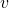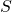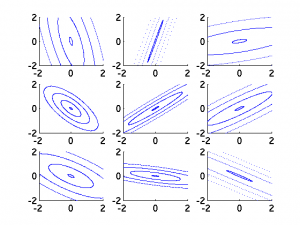# Inverse Wishart Distribution

The inverse Wishart distribution represents positive definite matrices.  This can be extremely useful in Bayesian analysis, as the inverse Wishart can serve as a prior for any covariance matrix.

## Basic Facts

Letbe a DxD positive definite matrix.  We sayis drawn from an inverse Wishart with parameters,when we have the following probability density functionwhere

•: scalar degrees of freedom
•: DxD positive definite matrix

The expected value (mean) ofunder this p.d.f. iswhich is only defined for. So for, we have, etc.

## Visualizations

To gain intuition about the influence of different parameters, here I show i.i.d. draws from an inverse Wishart with inverse scale matrixset to the 2-dimensional identity matrix. Each plot considers a different value of, the degrees of freedom.

Here, we use 2 degrees of freedom, the minimum permissible with a two-dimensional scale matrix.Moving to 8 degrees of freedom… we can see the shrinking size of the covariance matrixFinally, here’s 32 degrees of freedom.  There is very little variability here, as we’re converging to the mean (a scaled-down version of the identity matrix).